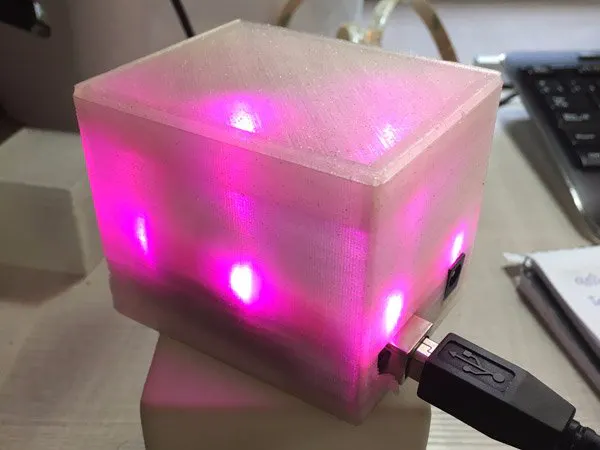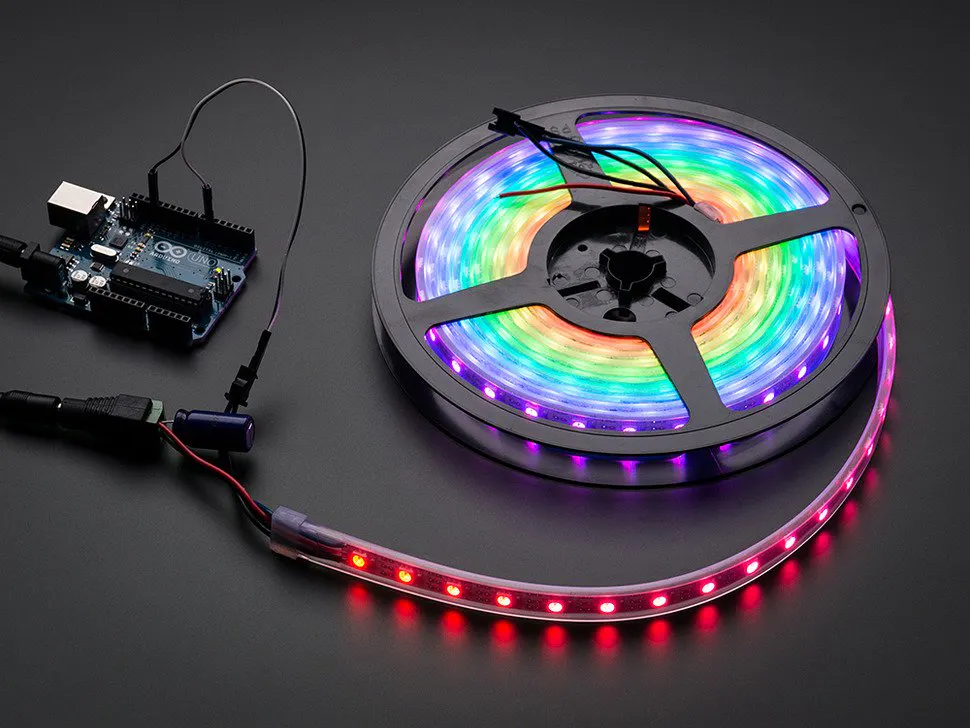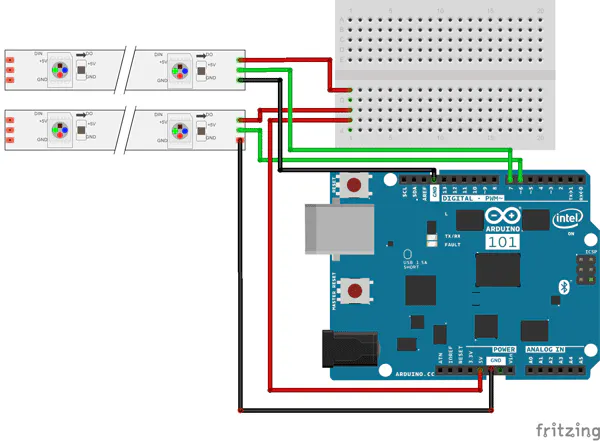# Motion Lamp with Arduino 101

Motion lamp is a prototype that allows controlling an RGB light lamp through movement with Arduino 101 and NeoPixel Strip.

IntermediateFull instructions provided2 hours3,512## Things used in this project

### Hardware componentsArduino 101
×1NeoPixel strip
×1

### Hand tools and fabrication machines3D Printer (generic)

## Custom parts and enclosures

### Cube 3D

This is the cube design we have used for this project.

## Schematics

### Motiom Lamp Schematics

Conections Arduino 101 with NeoPixel Strip## Code

### Motion Lamp with Arduino 101

Arduino
It is the main sketch where the whole logic of the project is implemented.
```#include <CurieIMU.h>
#include <RGBConverter.h>

#define PIN       6  // 11 pixels NeoPixel Strip
#define PIN1      7  // 1 pixel NeoPixel Strip
#define NUMPIXELS 11 // Numer of píxels
#define SAMPLE_RATE 25 // Sampling rate for accelerometer and gyroscope

float accelScale, gyroScale;

// NeoPixel configuration

// Color spaces
RGBConverter rgbConverter;
double h = 1;
double s = 1;
double v = 1;
byte rgb;

// Status Motion Lamp
// State 0 -> Select Hue - Pitch
// State 1 -> Select Saturation - Roll
// State 2 -> Select Value - Yaw
// State 3 -> Fix color
volatile int statusLamp = 0;

void setup() {
Serial.begin(9600);

// start the IMU and filter
CurieIMU.begin();
CurieIMU.setGyroRate(SAMPLE_RATE);
CurieIMU.setAccelerometerRate(SAMPLE_RATE);
filter.begin(SAMPLE_RATE);

// Set the accelerometer range to 2G
CurieIMU.setAccelerometerRange(2);
// Set the gyroscope range to 250 degrees/second
CurieIMU.setGyroRange(250);

CurieIMU.autoCalibrateAccelerometerOffset(X_AXIS, 0);
CurieIMU.autoCalibrateAccelerometerOffset(Y_AXIS, 0);
CurieIMU.autoCalibrateAccelerometerOffset(Z_AXIS, 1);
CurieIMU.autoCalibrateGyroOffset();

CurieIMU.attachInterrupt(eventCallback);
CurieIMU.setDetectionThreshold(CURIE_IMU_TAP, 950);
CurieIMU.interrupts(CURIE_IMU_TAP);

// initialize variables to pace updates to correct rate
microsPrevious = micros();

// Init NeoPixel 11
pixels.begin();
pixels.show();

// Init NeoPixel 1
pixelsStatus.begin();
pixels.show();

// Show status in px
setStatusPixel(statusLamp);
}

void loop() {
int aix, aiy, aiz; //accelerometer
int gix, giy, giz;
float ax, ay, az;
float gx, gy, gz;
float roll, pitch, yaw;
static unsigned long microsNow;

// check if it's time to read data and update the filter
microsNow = micros();
if (microsNow - microsPrevious >= microsPerReading) {

// read raw data from CurieIMU
CurieIMU.readMotionSensor(aix, aiy, aiz, gix, giy, giz);

// convert from raw data to gravity and degrees/second units
ax = convertRawAcceleration(aix);
ay = convertRawAcceleration(aiy);
az = convertRawAcceleration(aiz);
gx = convertRawGyro(gix);
gy = convertRawGyro(giy);
gz = convertRawGyro(giz);

// update the filter, which computes orientation
filter.updateIMU(gx, gy, gz, ax, ay, az);

// print the heading, pitch and roll
roll = filter.getRoll();
pitch = filter.getPitch();
yaw = filter.getYaw();

// increment previous time, so we keep proper pace

// Only if change Hue, Saturation or Value
if (statusLamp < 3)
{
// pitch only -90º to 90º = 180º
// State 0 -> select Hue
if (pitch >= -90 && pitch <= 90 && statusLamp == 0)
{
// Transform angle
pitch = pitch + 90;
// Obtains color cordinates from angles
h = pitch / 180.0;
}

// Angles restrictions
// roll only -90º to 90º = 180º
// State 1 -> select Saturation
if (roll >= -90 && roll <= 90 && statusLamp == 1)
{
// Transform angle
roll = roll + 90;
// Obtains color cordinates from angles
s = roll / 180.0;
}

// State 2 -> select Value
if (statusLamp == 2)
{
// yaw 0º to 360º
v = yaw / 360.0;
}

// Convert to rgb
rgbConverter.hsvToRgb(h, s, v, rgb);

/*
Serial.print("Color: ");
Serial.print(h);
Serial.print(" - ");
Serial.print(s);
Serial.print(" - ");
Serial.print(v);
Serial.println(" ");

Serial.print("Orientation: ");
Serial.print(yaw);
Serial.print(" ");
Serial.print(pitch);
Serial.print(" ");
Serial.println(roll); */

// Change color of the pixels
for (int px = 0; px < NUMPIXELS; px++)
{
pixels.setPixelColor(px, pixels.Color(rgb, rgb, rgb));
pixels.show();
}
}

// Show status in px
setStatusPixel(statusLamp);
}
}

float convertRawAcceleration(int aRaw) {
// since we are using 2G range
// -2g maps to a raw value of -32768
// +2g maps to a raw value of 32767

float a = (aRaw * 2.0) / 32768.0;
return a;
}

float convertRawGyro(int gRaw) {
// since we are using 250 degrees/seconds range
// -250 maps to a raw value of -32768
// +250 maps to a raw value of 32767

float g = (gRaw * 250.0) / 32768.0;
return g;
}

static void eventCallback()
{
// Detect tap in all axis
if (CurieIMU.getInterruptStatus(CURIE_IMU_TAP)) {
Serial.print("Tap detected statusLamp: ");
Serial.println(statusLamp);

// Change state
statusLamp++;

// Init state
if (statusLamp > 3)
{
statusLamp = 0;
}
}
}

void setStatusPixel(int statusPx)
{
switch (statusPx)
{
case 0:
pixelsStatus.setPixelColor(0, pixelsStatus.Color(150, 0, 0));
pixelsStatus.show();
break;
case 1:
pixelsStatus.setPixelColor(0, pixelsStatus.Color(0, 150, 0));
pixelsStatus.show();
break;
case 2:
pixelsStatus.setPixelColor(0, pixelsStatus.Color(0, 0, 150));
pixelsStatus.show();
break;
case 3:
pixelsStatus.setPixelColor(0, pixelsStatus.Color(0, 0, 0));
pixelsStatus.show();
break;

}
}
```

### CurieIMU

Library to obtain information from the IMU unit. We use it to get the motion sensor and to detect the tap.

Library to get the Euler angles and determine the cube spatial position.

This library controls the NeoPixel Strip.

### RGBConverter

This library converts between HSV and RGB color spaces.

## Credits

### Germán Martín

2 projects • 3 followers